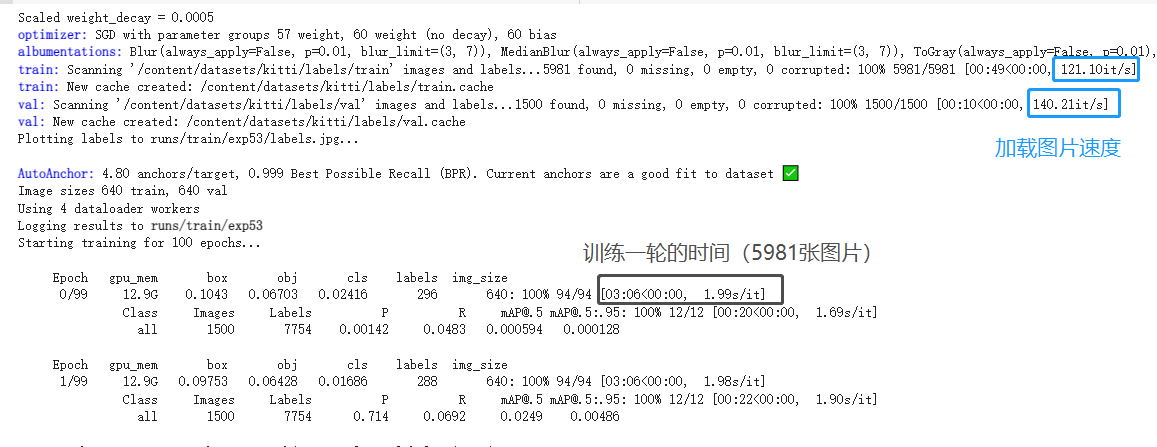[En]

## 思路1——直接把数据集放到Colab 中存储

``````!mkdir datasets
!ls
``````## 思路2——hdf5方式内存映射文件

HDF5 优点：它的层次结构（类似于文件夹/文件）、与每个项目一起存储的可选的任意元数据；以及它的灵活性（例如压缩）；另一个优点是数据集可以是固定大小的 _或_灵活的尺寸。因此，可以轻松地将数据附加到大型数据集，而无需创建全新的副本。

### 1、挂载谷歌网盘

``````from google.colab import drive
drive.mount('/content/drive')
``````

### 2、更新数据

``````!google-drive-ocamlfuse -cc
``````

## 3、hdf5方式映射数据集

Python 提供了 h5py 和 lmdb 库来处理这些文件格式。h5py 可以轻松地以 NumPy 数组的形式存储和操作现有数据，我们使用hdf5内存映射文件格式。

``````import h5py
from PIL import Image

fileName = 'data.h5'
numOfSamples = 10000
with h5py.File(fileName, "w") as out:
out.create_dataset("X_train",(numOfSamples,256,256,3),dtype='u1')
out.create_dataset("Y_train",(numOfSamples,1,1),dtype='u1')
out.create_dataset("X_dev",(numOfSamples,256,256,3),dtype='u1')
out.create_dataset("Y_dev",(numOfSamples,1,1),dtype='u1')
out.create_dataset("X_test",(numOfSamples,256,256,3),dtype='u1')
out.create_dataset("Y_test",(numOfSamples,1,1),dtype='u1')
``````

out.create_dataset("X_train",(numOfSamples,256,256,3),dtype='u1') 这行代码是将训练图像加载到 `X_train`"关键字"，图片宽高分别是256x256，3通道RGB。

``````with h5py.File(fileName, "a") as out:
img = Image.open("X_train_1.jpg")      # X_train_1.jpg is 256 x 256 RGB image
out['X_train'] = numpy.asarray(img)
``````

``````import torch
import numpy as np
import torch.optim as optim
import torch.utils.data
import torchvision.datasets as dset
import torchvision.transforms as transforms
import torchvision.utils as vutils
from PIL import Image
import h5py

class dataset_h5(torch.utils.data.Dataset):
def __init__(self, in_file, transform=None):
super(dataset_h5, self).__init__()

self.file = h5py.File(in_file, 'r')
self.transform = transform

def __getitem__(self, index):
x = self.file['X_train'][index, ...]
y = self.file['Y_train'][index, ...]

# Preprocessing each image
if self.transform is not None:
x = self.transform(x)

return (x, y), index

def __len__(self):
return self.file['X_train'].shape
``````

``````dataset = dataset_h5("PATH_TO_YOUR_.h5_FILE",transform=transform)
dataset, batch_size=8,
drop_last=True, shuffle=bshuffle, num_workers=1)
``````

Original: https://blog.csdn.net/qq_41204464/article/details/121734447
Author: 一颗小树x

Original: https://blog.csdn.net/qq_41204464/article/details/121734447
Author: 一颗小树x

## YOLO V6系列(二) — 网络结构解析

YOLO V6系列(二) – 网络结构解析 在YOLO V6系列(一) – 跑通YOLO V6算法这篇blog中简单的介绍了YOLO V6算法的训练及测试过程。那么后面，尽可能地对源码进行解析。首先，...